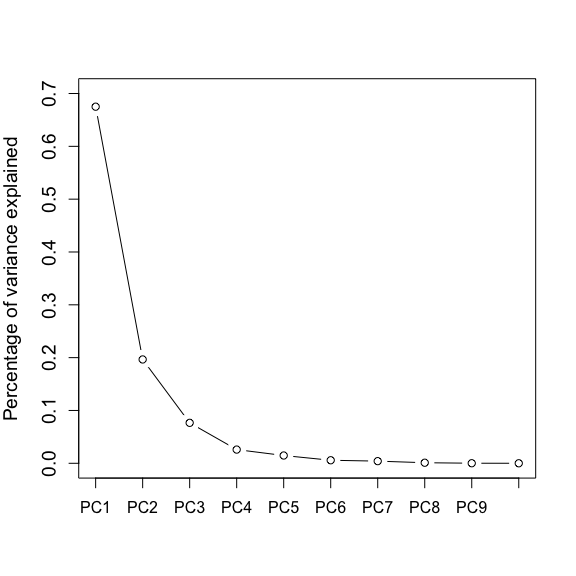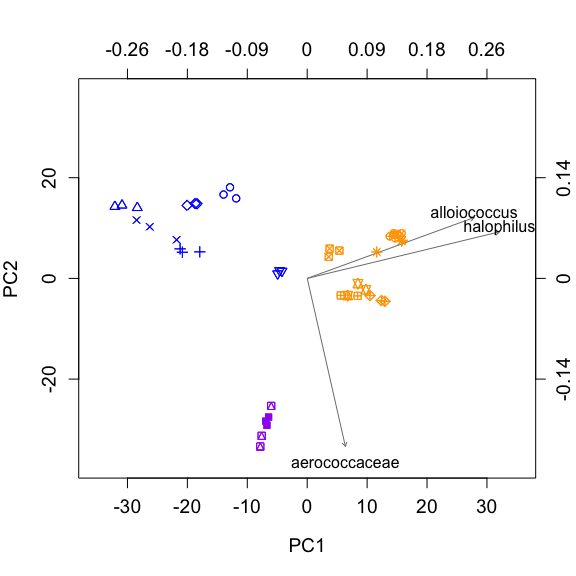# A tutorial for constructing a GMD-biplot

## Introduction

In this vignette, we illustrate how to construct the GMD-biplot and screeplot using the tobacco data set from (Satten et al. 2017). This data set includes 15 smokeless tobacco products: 6 dry snuffs, 7 moist snuffs, and 2 toombak samples from Sudan. Three separate (replicate) observations (starting with sample preparation) were made of each product, so that in total 45 observations are available. Each observation has a 271 × 1 vector of taxon counts. To make the measurements comparable, we consider the centered log ratio (CLR) transformation of the data set. Additionally, the squared weighted UniFrac distance, denoted $$\Delta$$, is used to measure the distance between samples. The corresponding similarity kernel $$H$$ is derived from $$\Delta$$ using the Gower’s centering matrix.

We first load our R package GMDecomp.

library(GMDecomp)

The data object tobacco_clr in the package include

• data: the CLR transformed out table, with rows for samples and columns for OTU.
• $$H$$: the similarity kernel derived from the squared weighted UniFrac distance.
• sample.col: the color for plotting each sample point.
• sample.pch: the shape for plotting each sample point.
• out.names: the taxonomic name of each OTU.

## Step 2: Generalized Matrix Decomposition

To construct the GMD-biplot and screeplot, we need to first perform the generalized matrix decomposition (Allen, Grosenick, and Taylor 2014) of the data with respect to $$H$$. This can be easily achieved using the following line:

tobacco.gmd <- GMD(X = tobacco_clr$data, H = tobacco_clr$H, Q = diag(1, dim(tobacco_clr$data)), K = 10) Note that here we don’t have a similarity kernel for the OTUs, so $$Q$$ is set to be an identity matrix. One can set $$Q$$ to be any informative positive semi-definite matrix, if such information is available. Also, here we set $$K = 10$$, since we want to display the screeplot the top 10 GMD components. If only the GMD-biplot is needed, one can set $$K = 2$$, which may save computational time. tabacco.gmd is a list of class gmd, which consists of the following variables. • U: the left GMD components with 45 rows and 10 columns. • S: the top 10 GMD values. • V: the right GMD components with 271 rows and 10 columns. • H: the similarity matrix for samples. • Q: the similarity matrix for OTUs. ## Step 3: The GMD-biplot and screeplot Once the GMD outputs are obtained, the screeplot can by easily constructed as follows. screeplot(tobacco.gmd) #the screeplot of the top 10 GMD componentsNote that one can select specific OTUs to display in the GMD-biplot. For this analysis, we display the top 3 OTUs that have the longest arrows. gmd.order = order(rowSums(tobacco.gmd$V[,1:2]^2), decreasing = T)
plot.index = gmd.order[1:3]
plot.names = tobacco_clr$otu.names[plot.index] biplot(fit = tobacco.gmd, index = plot.index, names = plot.names, sample.col = tobacco_clr$sample.color, sample.pch = tobacco_clr\$sample.pch, arrow.col = 'grey50')